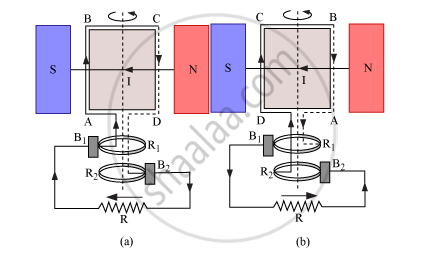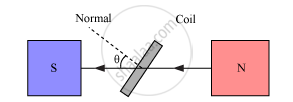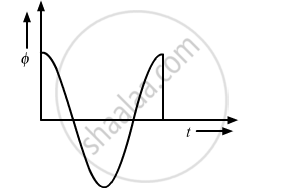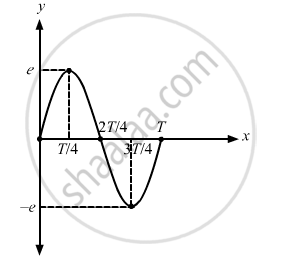# Draw a Schematic Sketch of an Ac Generator Describing Its Basic Elements. State Briefly Its Working Principle. - Physics

Draw a schematic sketch of an ac generator describing its basic elements. State briefly its working principle. Show a plot of variation of

(i) Magnetic flux and

(ii) Alternating emf versus time generated by a loop of wire rotating in a magnetic field.

#### Solution

Principle − Based on the phenomenon of electromagnetic induction.

Construction:Main parts of an ac generator:

Armature − The rectangular coil ABCD

Filed Magnets − Two pole pieces of a strong electromagnet

Slip Rings − The ends of the coil ABCD are connected to two hollow metallic rings R1 and R2.

Brushes − B1 and B2 are two flexible metal plates or carbon rods. They are fixed and are kept in tight contact with R1 and R2, respectively.

Working − As the armature coil is rotated in the magnetic field, angle θ between the field and the normal to the coil changes continuously. Therefore, magnetic flux linked with the coil changes and an emf is induced in the coil. According to Fleming’s right hand rule, current is induced from A to B in AB and from C to D in CD. In the external circuit, current flows from B2 to B1.

To calculate the magnitude of emf induced:

Suppose  A → Area of each turn of the coil

N → Number of turns in the coil

vecB→ Strength of the magnetic field

θ → Angle which normal to the coil makes with vecB at any instant t∴ Magnetic flux linked with the coil in this position is given by,

phi=N(vecB.vecA)=NBAcostheta=NBAcos

Where, ‘ω’ is angular velocity of the coil

Graph between magnetic flux and time, according to equation (i), is shown below:As the coil rotates, angle θ changes. Therefore, magnetic flux Φ linked with the coil changes and an emf is induced in the coil. At this instant t, if e is the emf induced in the coil, then

e=(dtheta)/dt=-d/dt(NABcos

=-NABd/dt(cos

=-NAB(-sin ωt)ω

∴ e = NAB ω sin ωt

The graph between alternating emf versus time  is shown below:Concept: Magnetic Flux
Is there an error in this question or solution?
2013-2014 (March) Delhi Set 1

Share